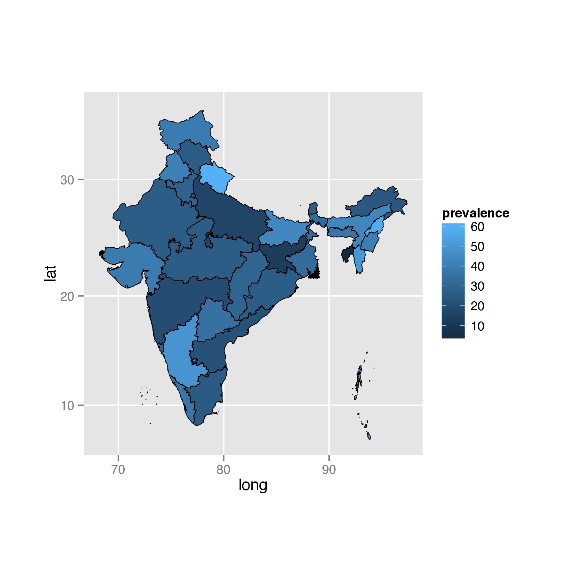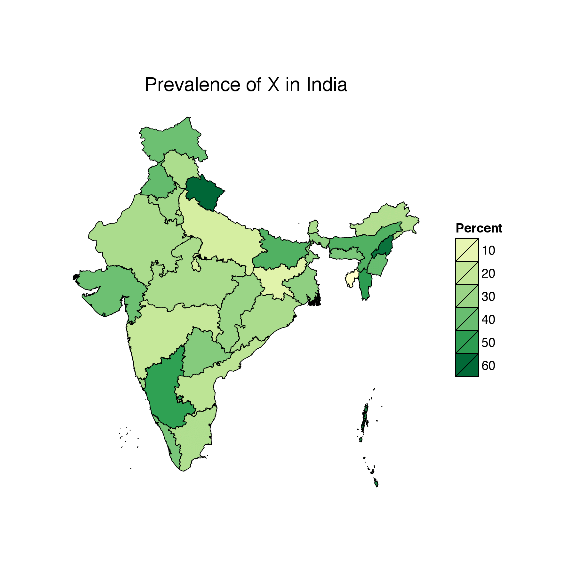## Tuesday, October 27, 2015

### Mapping with ggplot: Create a nice choropleth map in R

I was working on making a map in R recently and after an extensive search online, I found a hundred different ways to do it and yet each way didn’t work quite right for my data and what I wanted to do. Eventually, I found a way to make it work after pulling together code from this blog, this blog, and this stack overflow page. Combining all of those sources, along with a good amount of trial and error, has led to this blog post.

This post shows how to use ggplot to map a choropleth map from shapefiles, as well as change legend attributes and scale, color, and titles, and finally export the map into an image file.

### Preparing the data

We need a number of packages to make this work, as you can see below so make sure to install and load those. We load in shapefiles using the readShapeSpatial() function from the maptools library. A shapefile is a geospatial vector data storage format for storing map attributes like latitude and longitude. You can download lots of shape files for free! For example, for any country in the world you can download shape files here. Or just google it.

My project is to graph some data on India so I have downloaded a shapefile with state boundaries of India and I will now load that into R.

``````library(ggplot2)
library(maptools)
library(rgeos)
library(Cairo)
library(ggmap)
library(scales)
library(RColorBrewer)
set.seed(8000)

##set directory to the folder where the shapefile is, then input shapefile
class(states.shp)``````
``````##  "SpatialPolygonsDataFrame"
## attr(,"package")
##  "sp"``````
``names(states.shp)``
``````##  "ID_0"      "ISO"       "NAME_0"    "ID_1"      "NAME_1"    "TYPE_1"
##  "ENGTYPE_1" "NL_NAME_1" "VARNAME_1"``````

The class of the loaded shapefiles are of a spatial class, and we can see what is in it by checking its names. You can print out these components to see what they are. For example, let’s print out the state names like this:

``print(states.shp\$NAME_1)``
``````##   Andaman and Nicobar    Andhra Pradesh         Arunachal Pradesh
##   Assam                  Bihar                  Chandigarh
##   Chhattisgarh           Dadra and Nagar Haveli Daman and Diu
##  Delhi                  Goa                    Gujarat
##  Haryana                Himachal Pradesh       Jammu and Kashmir
##  Jharkhand              Karnataka              Kerala
##  Manipur                Meghalaya              Mizoram
##  Nagaland               Orissa                 Puducherry
##  Punjab                 Rajasthan              Sikkim
##  Tamil Nadu             Telangana              Tripura
##  Uttar Pradesh          Uttaranchal            West Bengal
## 36 Levels: Andaman and Nicobar Andhra Pradesh Arunachal Pradesh ... West Bengal``````

Now we’ll get the data we want to plot on the map. Here I’ll just make the data up, but you can import a csv of it or extract it from your model estimates. The important thing is that the id numbers are the same in the shape file as in your data you want to plot, because that will be necessary for them to merge properly (you could also merge by the name itself).

``````##create (or input) data to plot on map
num.states<-length(states.shp\$NAME_1)
mydata<-data.frame(NAME_1=states.shp\$NAME_1, id=states.shp\$ID_1, prevalence=rnorm(num.states, 55, 20))
``````##                NAME_1 id prevalence
## 1 Andaman and Nicobar  1   80.84018
## 2      Andhra Pradesh  2   36.94596
## 3   Arunachal Pradesh  3   40.57686
## 4               Assam  4   67.05668
## 5               Bihar  5   67.23842
## 6          Chandigarh  6   44.20251``````

Important Note: ID_1 is the ID that uniquely identifies that state in this shapefile so that is what I’m using in my prevalence data and that is what I use when I fortify in the next paragraph. You should look at your shapefiles and determine what ID uniquely determines the data and use that (for example, it may be ID_2). This ID must also be the SAME ID as in the mapping data (what is called here mydata). Please do this to check:

``print(mydata\$id)``
``````##    1  2  3  4  5  6  7  8  9 10 11 12 13 14 15 16 17 18 19 20 21 22 23
##  24 25 26 27 28 29 30 31 32 33 34 35 36``````
``print(states.shp\$ID_1)``
``````##    1  2  3  4  5  6  7  8  9 10 11 12 13 14 15 16 17 18 19 20 21 22 23
##  24 25 26 27 28 29 30 31 32 33 34 35 36``````

If those are not exactly the same (and unique), you will encounter an error later on. Please change your mydata\$id to match the states.shp ID you are using.

Now we need to merge the shapefile and the dataset together. First, we fortify() the shapefile (a function of ggplot) to get it into a dataframe. We need to include a region identifier so that ggplot keeps that id when it turns it into a dataframe; otherwise it will make it up and it will not be possible to merge properly. This should be the same region identifier as what is in your prevalence data (or that data you want to map) so that later this can be merged properly.s

``````#fortify shape file to get into dataframe
states.shp.f <- fortify(states.shp, region = "ID_1")
class(states.shp.f)``````
``##  "data.frame"``
``head(states.shp.f)``
``````##       long      lat order  hole piece id group
## 1 92.89889 12.91583     1 FALSE     1  1   1.1
## 2 92.89917 12.91555     2 FALSE     1  1   1.1
## 3 92.89943 12.91556     3 FALSE     1  1   1.1
## 4 92.90000 12.91500     4 FALSE     1  1   1.1
## 5 92.90028 12.91528     5 FALSE     1  1   1.1
## 6 92.90056 12.91528     6 FALSE     1  1   1.1``````

We can see that the class is now a common dataframe and each row is a longitude and latitude.

Now we can merge the two dataframes together by the id variable, making sure to keep all the observations in the spatial dataframe. Importantly, we need to order the data by the “order” variable. You can see what happens if you don’t do this and it’s not pretty.

``````#merge with coefficients and reorder
merge.shp.coef<-merge(states.shp.f, mydata, by="id", all.x=TRUE)
final.plot<-merge.shp.coef[order(merge.shp.coef\$order), ] ``````

### Basic plot

We’re ready to plot the data using ggplot(), along with geom_polygon() and coord_map(). Note that in the aes statement, group=group does NOT change; “group” is a variable in the fortified dataframe so just leave it.

You do need to change “prevalence” to whatever variable you want to plot.

``````#basic plot
ggplot() +
geom_polygon(data = final.plot,
aes(x = long, y = lat, group = group, fill = prevalence),
color = "black", size = 0.25) +
coord_map()``````Troubleshooting: I have had many comments about an error occuring at this step, with usually this error:

Error in seq.default(from = best\(lmin, to = best\)lmax, by = best\$lstep) : ‘from’ must be of length 1

If you receive this error, that means that the shapefile did not merge properly with the mapping data. Go back to the Important Note above, and follow the instructions for making sure you have unique and matching IDs across your shapefile and mapping data.

### Making the map pretty

This is a great start, but there are a number of options that can make the map look much nicer. First, we can remove the background and gridlines by including theme_nothing() from the ggmap package, but indicating that we still want the legend. We can also change the title of the legend and add in a title for the whole map.

Next, we can change the palette to “YlGn” which is Yellow to Green. You can find palette options as well as help on color in R here, or type in display.brewer.all() to see a plot of them in R.

We can also use pretty_breaks() from the scales package to get nice break points (the pretty algorithm creates a sequence of about n+1 equally spaced round values, that are 1, 2, or 5 times a power of 10). If you don’t like the way pretty_breaks looks, you can change your scale with scale_fill_gradient() and set the limits and colors like this:

scale_fill_gradient(name=“My var”, limits=c(0,100), low=“white”, high=“red”) I do this in the third map, below.

``````#nicer plot
ggplot() +
geom_polygon(data = final.plot,
aes(x = long, y = lat, group = group, fill = prevalence),
color = "black", size = 0.25) +
coord_map()+
scale_fill_distiller(name="Percent", palette = "YlGn", breaks = pretty_breaks(n = 5))+
theme_nothing(legend = TRUE)+
labs(title="Prevalence of X in India")``````Other options:

If we wanted to reverse the color to go from 0 being dark instead of light, we can just add in the option trans=“reverse” into the scale_fill_distiller statement (see the plot below).

If we were plotting a discrete variable instead of a continuous one, we would use scale_fill_manual instead of distiller, like this:

scale_fill_manual(name=“My discrete variable”, values=c(“red”, “blue”, “green”, “yellow”))

Additionally, we can put the names of the states on the map. This may or may not be a great idea depending on the size of the areas you’re mapping, but if it’s useful, you can do it easily using geom_text(). First we need to identify a longitude and latitude where you want to plot each state name. One way we can do this is using aggregate() to calculate the mean of the range of the longitude and latitude for each state.

``````#aggregate data to get mean latitude and mean longitude for each state
cnames <- aggregate(cbind(long, lat) ~ NAME_1, data=final.plot, FUN=function(x) mean(range(x)))

#plot using geom_text
ggplot() +
geom_polygon(data = final.plot,
aes(x = long, y = lat, group = group, fill = prevalence),
color = "black", size = 0.25) +
coord_map() +